# Point-Slope Equation of a Line

### The "point-slope" form of the equation of a straight line is:

y − y1 = m(x − x1)

The equation is useful when we know:

• one point on the line: (x1,y1)
• and the slope of the line: m,

and want to find other points on the line.

Have a play with it first (move the point, try different slopes):

Now let's discover more.

## What does it stand for?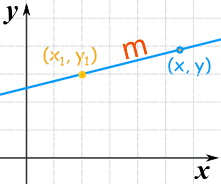(x1, y1) is a known point

m is the slope of the line

(x, y) is any other point on the line

## Making sense of it

It is based on the slope: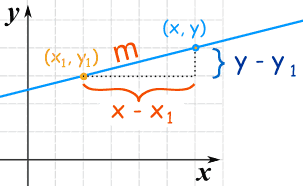Slope m  =   change in y change in x   =   y − y1 x − x1

 Starting with the slope: we rearrange it like this:   to get this: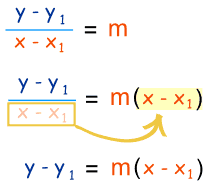So, it is just the slope formula in a different way!

### Example 1: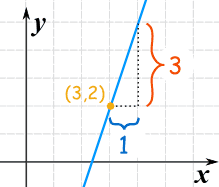slope "m"  =  31  =  3

y − y1 = m(x − x1)

We know m, and also know that (x1, y1) = (3,2), and so we have:

y − 2 = 3(x − 3)

That is a perfectly good answer, but we can simplify it a little:

y − 2 = 3x − 9

y = 3x − 9 + 2

y = 3x − 7

### Example 2:m = −3 1 = −3

y − y1 = m(x − x1)

We can pick any point for (x1, y1), so let's choose (0,0), and we have:

y − 0 = −3(x − 0)

Which can be simplified to:

y = −3x

### Example 3: Vertical Line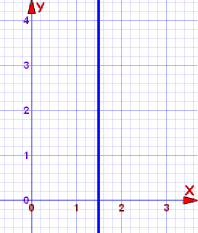What is the equation for a vertical line?
The slope is undefined!

In fact, this is a special case, and we use a different equation, like this:

x = 1.5

Every point on the line has x coordinate 1.5,
that’s why its equation is x = 1.5

## What About y = mx + b ?

You may already be familiar with the "y=mx+b" form (called the slope-intercept form of the equation of a line).

It is the same equation, in a different form!

The "b" value (called the y-intercept) is where the line crosses the y-axis.

So point (x1, y1) is actually at (0, b)

and the equation becomes: